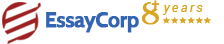WhatsApp
Struggling With Assignments?

We are here to help.

Chat with our expert writers on WhatsApp

for any kind of assignment queries.

Click to chat!
Struggling with Assignments? WhatsApp UsFew tasks related to different cipher processes, from ancient Caesar cipher to digital signature of files. This is a great way to practice and fully comprehend concepts of hash, public key, private key and cracking and encryption. In this case we based some of the task on Linux commands and the rest required only simple math calculations

1.Introduction

In this activity we did some tasks related to different cipher processes, from ancient Caesar cipher to digital signature of files. This is a great way to practice and fully comprehend concepts of hash, public key, private key and cracking and encryption. In this case we based some of the task on linux commands and the rest required only simple math calculations.

My student number is 3408050 where the number module 10 is 9 and it was calculated as it follows: (3+4+0+8+0+5+0) = 20 , then 20 /10 has a remainder of 0. By using the Caesar cipher with the known shift 0 the alphabet abcdefghijklmnopqrstuvwxyz results abcdefghijklmnopqrstuvwxyz because the shift is null. I is the correct answer according to the instructions but the result does not gives a good example of a Caesar cipher. This is why I created the folder Task11_B because in this case I decided to sum the student number in this sequence 3+40+80+50 = 173, where 173/10 has a remainder of 3. Now with a shift number of 3 the Caesar cipher of abcdefghijklmnopqrstuvwxyz results defghijklmnopqrstuvwxyzabc. So the lastname Kubay is cipher as nxedbf.

In order to create the sha256 hash and save it on the file we proceed with this linux command concatenation: echo -n 3408050 | sha256sum | awk '{print \$1}' >3408050_Task_12_hash.txt Now we can encrypt this has using the public key (sicpub.key) using this command: cat 3408050_Task_12_hash.txt | openssl rsautl -encrypt -pubin -inkey sicpub.key > 3408050_Task _12.txt

We proceed to create the private key with this command: openssl genpkey -algorithm RSA -out 3408050pri.key -pkeyopt rsa_keygen_bits:2048 Then we have to create the public key from the private key: openssl rsa -pubout -in 3408050pri.key -out 3408050pub.key Now we sign the file 3408050_Task_12_hash.txt with the private key and output the file 3408050_Task_13.txt: openssl rsautl -sign -inkey 3408050pri.key -out 3408050_Task_13.txt -in 3408050_Task_12_hash.txt We can check if the file 3408050_Task_13.txt is correct with the public key: openssl rsautl -verify -inkey 3408050pub.key -keyform PEM -in 3408050_Task_13.txt -pubin The result must be the hash decripted.

6.Conclusion

It is very important to distinguish between a symmetric and asymmetric cipher as well as to understand when is useful to use a hash, when we need to sign digital a file or when do we need to encrypt it. By doing this tasks we have the opportunity to familiarize with this techniques to make data more secure.

HIRE EXPERT
Email ID *
Subject
Pages